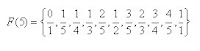## Saturday, 13 December 2008

### A Curious Property of Vulgar FractionsJohn Farey was a geologist, not a mathematician, but he is better rememberd today for a single short (four paragraphs) paper he wrote in 1816 than for all his good works in geology. In that year he sent a paper to the Philosophical Magazine (or at least it was published in that year) called On a curious property of vulgar fractions .and described a pattern that appears in sequences of what we would today call common (vulgar is the latin term for common) fractions, like 3/8 etc, which are in simplest terms. They observation has almost NO practical use, not even to prove other things mathematically, and yet, it seems to have all kinds of interesting properties that tend to keep us fascinated with it. If you have never been introduced to it, here is a brief description, and some novel relationships that I think are interesting, with some links to places where they are made clearer than I could do in this brief space.

So First... What is it we are talking about? If you take ALL the fractions that could be written in simplest terms with a denominator less than some number n, say n=5 (since that is the one Farey used in his paper), and put them in order from lowest to highest... you getThe "curious" thing that Farey noticed is that if you ignore the way your fifth grade teacher taught you to add fractions, and do it the way YOU would have added them, "add the tops, add the bottoms", then each number in the sequence is the sum of the terms on each side of it... for example 1/4 and 2/5 are on each side of 1/3, and if you add by this approach you get (1+2)/(4+5) = 3/9 and that simplifies to 1/3 . The number obtained by adding two fractions in this fashion is often called the mediant .

OK, so that is how you make them. Our first question might be, how many of them are there? F(5) obviously has eleven terms (I counted). If we picked a value of N, what would be the number of fractions in the set F(N). A little investigation would show that F(1) = 2 (0 and 1); and F(2) = 3 (0, 1/2, and 1). So how many would be added to the next set... and the next... it turns out that each new set will have all the values of the previous set (of course) and will add one for every value of one through n that is co-prime (has no common divisors) to n. So the set for N=6 will have the eleven terms of F(5) plus 1/6 (one has no common factor with six), and 5/6. (notice that 2/6, 3/6, and 4/6 are already in the sequence in F(5) in simplified form)... thus F(6) has thirteen terms.. and in general we get a recursive formula that say the Order of F(N) = Order of F(n-1) + φ(n).

A second nice curiosity related to Farey Sequences are the Ford Circles. "Ford circles are named after American mathematician Lester R. Ford, Sr., who described them in an article in American Mathematical Monthly in 1938, volume 45, number 9, pages 586-601" (from Wikipedia). In fact, the
wikipedia article is a nice place to see how they work, and I need say no more as it shows the circles for F(5). What is amazing is that each circle is tangent to every other circle for a fraction it will be adjacent to in ANY sequence.... ahhh, go on..say "cool".

I decided to mention this when I came across another curious property of Farey sequences that relate them to lines on the plane and Pick's Theorem. If you treated each fraction a/b as a point (b,a) then none of the lines cross. If you make a triangle with the origin and any two adjacent Farey fractions, since each of the triangles have a determinant of one (meaning the area is 1/2) and therefore, by PIck's theorem, they cannot contain any other lattice points in their interior. A nice explanation of this, including the photo below, is at the Cut-The-Knot web siteAnonymous said...
This comment has been removed by a blog administrator.Anonymous said...

It is really curious to see that such an important operation: 'Mediant', and its generalization called 'Rational Mean' have been always clasiffied as just 'Curious properties' and confined exclusively to specialized Number Theory books, however the general and unifying concept called 'Rational Mean' is much more than that, as you can see at:
http://mipagina.cantv.net/arithmetic/rmdef.htm

There you will find a section entitled: "The Freshman Problem" which I think could be of interest to you.
Best regards,

Domingo Gomez Morin
Structural Engineer.
Caracas
Venezuela